# Modular form

(diff) ← Older revision | Latest revision (diff) | Newer revision → (diff)

of one complex variable, elliptic modular form

A functionon the upper half-planesatisfying for some fixedthe automorphicity condition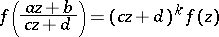(1)

for any element(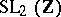is the group of integer-valued matrices with determinant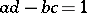), and such thatwhere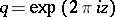,,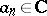. The integer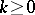is called the weight of the modular form. If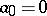, thenis called a parabolic modular form. There is also  a definition of modular forms for all real values of.

An example of a modular form of weightis given by the Eisenstein series (see )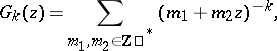where the asterisk means that the pairis excluded from summation. Herefor oddand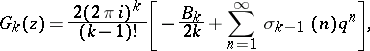whereand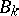is the-th Bernoulli number (cf. Bernoulli numbers).

The set of modular forms of weightis a complex vector space, denoted by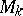; in this connection,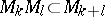. The direct sumforms a graded algebra isomorphic to the ring of polynomials in the independent variables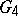and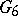(see ).

For each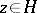the complex torus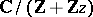is analytically isomorphic to the elliptic curve given by the equation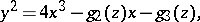(2)

where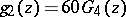,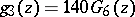. The discriminant of the cubic polynomial on the right-hand side of (2) is a parabolic modular form of weight 12: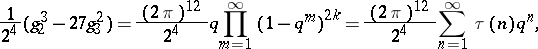where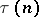is the Ramanujan function (see ).

For each integer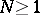modular forms of higher levelhave been introduced, satisfying (1) only for elementsof a congruence subgroupof levelof the modular group. In this case, related to the modular formis the holomorphic differential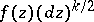on the modular curve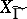. A well-known example of a modular form of higher level is the theta-seriesassociated to an integer-valued positive-definite quadratic form:which is a modular form of higher level and of weight. In this exampleis the integer equal to the number of solutions of the Diophantine equation.

The theory of modular forms allows one to obtain an estimate, and sometimes a precise formula, for numbers of the type(and congruences, such as the Ramanujan congruence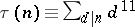(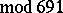)), and also to investigate their divisibility properties (see ). Best estimates for numbers of the type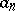have been obtained (see ).

Important arithmetic applications of modular forms are related to the Dirichlet seriesi.e. the Mellin transform of. Such Dirichlet series have been the subject of detailed study (estimates of coefficients, analyticity properties, the functional equation, Euler product expansion) in view of the presence of a non-trivial ring of correspondenceson a modular curve. For a curvethis ring is generated by the correspondence, whereruns through the set of all representatives of the elements of the quotient set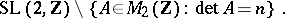The correspondences induce linear operators (Hecke operators) acting on the space of modular forms. They are self-adjoint relative to the Peterson scalar product (see , ). Modular forms which are eigen functions of the Hecke operators are characterized by the fact that their Mellin transforms have Euler product expansions.

Another direction in the theory of modular forms is related to the study of modular curves and the associated fibrations, the Kuga varieties (cf. Modular curve), and also to the theory of infinite-dimensional representations of algebraic adèle groups. Here the theory of modular forms of one variable was successfully transferred to the case of several variables (see ). A survey of the number-theoretic applications of modular forms is given in .

How to Cite This Entry:
Modular form. Encyclopedia of Mathematics. URL: http://encyclopediaofmath.org/index.php?title=Modular_form&oldid=13435
This article was adapted from an original article by A.A. Panchishkin (originator), which appeared in Encyclopedia of Mathematics - ISBN 1402006098. See original article